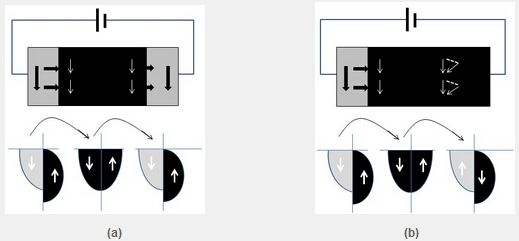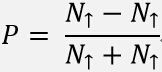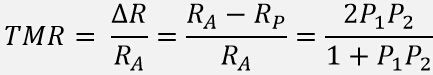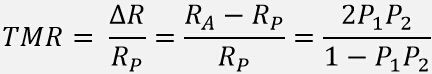MR Sensor Technology

MR Effect Physical Explanation

Date：03-12-2015    Hits：9851

Magnetoresistance of the magnetic tunnel junction (MTJ) also known as tunneling magnetoresistance (TMR) is a result of the spin-dependent tunneling effect.  The typical structure of an MTJ is two ferromagnetic layers separated by a thin insulating barrier (FM/I/FM).  This is sometimes referred to as a “sandwich structure.”  In sensor applications, one ferromagnetic layer is usually designed such that its magnetization does not move in response to an applied magnetic field.  This layer is often called the pinned layer. The other ferromagnetic layer is designed such that the magnetization moves easily in response to an applied magnetic field, and this layer is thus often called the free layer.  Using this basic concept, the relative orientation of the magnetization of the pinned and freelayers is representative of the strength and direction of an applied magnetic field.  Because the tunneling probability for electrons to cross the insulating barrier is dependent on the relative orientation of the free and pinned layer magnetizations, the resistance of an MTJ is indicative of the applied magnetic field.(a) Magnetization of two ferromagnetic layers are parallel to each other

(b) Magnetization of two ferromagnetic layers are anti-parallel to each other

Figure 1: Schematic diagram of the spin-dependent tunneling effect, the physical basis of the effect measured as TMR

As shown in Figure 1; when the magnetizations of the two ferromagnetic layers on either side of the tunnel barrier are parallel, the majority (up-arrow) electron subband on the top side matches the majority (up-arrow) electron subband on the bottom side, the minority (down-arrow) electron subband on the top side matches the minority (down-arrow) electron subband on the bottom side. This is the condition for maximum electron tunneling current (low resistance). When the magnetizations of the two ferromagnetic layers are antiparallel, the opposite is true: the electron subbands on either side of the barrier are mis-matched. The majority (up-arrow) electron subband on the top side does not match the majority (up-arrow) electron subband on the bottom side.  And the minority (down-arrow) electron subband on the top side does not match the minority (down-arrow) subband on the bottom side. This subband mismatch results in minimized electron tunneling current (high resistance). One can then design the ferromagnetic layers so that applying an external magnetic field changes the relative magnetization direction of the top and bottom ferromagnetic layers.  This changes the tunneling resistance, and is referred to as “TMR.”

In the two ferromagnetic layers in an MTJ, the electrode spin polarization is defined as:(1)

where N ↑ and N ↓ are, at the ferromagnetic metal Fermi level, the spin-up and spin-down, respectively, electron density of states.

Using the Julliere Model, it can be shown that:(2)

Or, alternatively:(3)

where RP and RA are, respectively the electrical resistances when the magnetization direction of the two ferromagnetic layers are parallel, and anti-parallel, to each other.  And P1and P2are, respectively, the electron spin polarizations in the two ferromagnetic layer electrodes. Obviously, if P1 and P2 are nonzero, the MTJ will exhibit the TMR effect. As the spin polarization of the two ferromagnetic layers electrodes increases, so too will the TMR value increase.

In the literature, different scholars have used different ways to define the TMR values. Some scholars use the definition in equation (2); but in recent years, most scholars have adopted the definition in equation (3).

•